# Project 2: Electromagnetic Waves

Imported and adapted from the UCLA Plasma Simulation Group's JupyterPIC Notebooks

Before anything else, we need to change to the stored notebook's directory, which contains input files for the PIC simulator.

cd /notebooks/light-wave-dispersion

In this project, we are going to look at the dispersion relation for electromagnetic waves.

The dispersion relation, $\omega(k)$, tells us the natural frequencies of oscillations for these waves, and the information contained in this function about the relationship between $\omega$ and $k$ can be used to determine the phase and group velocities of these waves. [There will be a subsequent notebook on wave velocities]

For transverse waves have:

• $\nabla \cdot \vec{E} = 0$ -- transverse waves

• $T_e = 0$ -- cold plasma

• $\vec{B}_0 = 0$ -- unmagnetized

From Maxwell's Equations we have:

and

Taking the curl of the first equation and substituting into it the second equation, we get:

Since $-\nabla\times\nabla\times\vec{A} = \nabla^2\vec{A} - \nabla(\nabla \cdot\vec{A})$, we have

For transverse waves, $\nabla \cdot\vec{E_1} = 0$, so

Where in the third line we used Euler's equations. Plugging in our definitions for $\Omega_p$ and $\omega_p$ and moving everything to the left hand side, we finally have

Note as in longitudinal waves in cold unmagnetized plasmas the term $[\Omega_p^2 + \omega_p^2]$ appears. As before, we note that this is a high frequency wave and hence approximate the ions as fixed due to their large mass, hence $[\Omega_p^2 + \omega_p^2] \simeq \omega_p^2$, and we write the above equation as

Next, assuming $\vec{E} = \vec{\bar{E}} e^{i(\vec{k} \cdot \vec{x} - \omega t)}$, we finally obtain

the dispersion relation for an electromagnetic wave in an unmagentized plasma!

It is plotted below.

# Plotting w(k)
import numpy as np
import matplotlib.pyplot as plt
%matplotlib inline

N = 5
k = np.linspace(-N,N,N*20)
w_p = 1
c = 1
w = np.sqrt(w_p**2 + c**2 * k**2)
cline = k
plt.plot(k,w, label='$\omega$(k)')
plt.plot(k,cline, label='slope = c')
plt.xlabel('k [$c/\omega_p$]')
plt.ylabel('$\omega$ (in units of $\omega_p$)')
plt.xlim(-N,N)
plt.ylim(0,N+1)
plt.grid(b=True, which='major', axis='both')
plt.legend(loc=0)
plt.show()• recalling expressions for phase velocity, $v_{\phi}=\omega/k$, and group velocity, $v_g = \frac{d\omega}{dk}$ we obtain
• Comparing this with the plot, confirm that indeed $v_g = 0$ for $k = 0$, and that $v_g \rightarrow c$ as $k \rightarrow \infty$. Importantly, although $v_{\phi} > c$, we have $v_g < c$. Thus special relativity is not violated, since information can only propagate at the group velocity and not at the phase velocity.

• Also note, if $\omega < \omega_p$ a wave cannot propogate since $k$ becomes imaginary and we get an evanescent wave.

## 1. Simulations with a Particle-in-Cell Code

In this project you simulate plasmas with exactly the same conditions as in Project 1a.

Each plasma electron is initialized with positions (only in $x$ or what we call $x_1$) such that the density is uniform. The ions are initialized at the same positions but they have an infinite mass. Each electron is also initialized with velocities ($v_1$, $v_2$, $v_3$) or momentum ($mv_1$, $mv_2$, $mv_3$) from a Maxwellian in each direction. The particles then begin to move in the self-consistent fields that their current and charge density produce.

• The length of the plasmas is 50 $c/\omega_p$
• The simulation will run for a time 400 $1/\omega_p$.
• The simulation uses 50,000 particles.

You will be looking at plots of the electric field in the $x_3$ direction, $E_3$. In Project 1a you plotted $E_1$.

### 1.1. The following lines must always be executed before running anything else.

Reminder: Hit Shift+Enter to run a cell, or select the cell and click on the "Run" button in the top menu bar

import osiris
%matplotlib inline

## 2. Run a case in which  $vth_1=vth_2=vth_3=0.02 c$.

# vth/c = 0.02
dirname = 'v02'
osiris.runosiris(rundir=dirname,inputfile='v02.txt')

After the simulation is finished, plot $E_3(x_1)$ at $t \approx 100$ (run the next cell).

• Do you see any evidence of an electromagnetic wave?
• Does the plot make sense?
dirname = 'v02'
osiris.field(rundir=dirname, dataset='e3', time=100)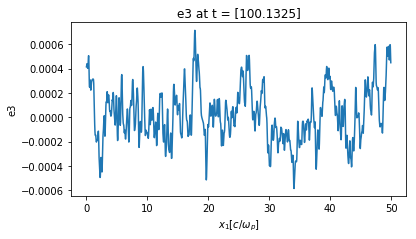Next, plot $E_3(t)$ at $x_1=5 c/\omega_p$ (i.e., at cell=100).

• Do you see any evidence of an electromagnetic wave?
• Does the plot make sense?
dirname = 'v02'
osiris.field(rundir=dirname, dataset='e3', space=100)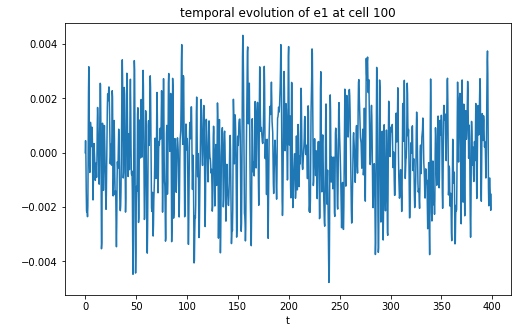Next, in the following two cells, we are going to plot $\omega(k)$. This is generated by taking $E_3(x_1,t)$ and Fourier analyzing in both position and time.

• $\omega(k)$ with wavenumber in units of [k] = $\omega_{pe}/c$:
dirname = 'v02'
osiris.plot_wk(rundir=dirname, wlim=[0,10], klim=[0,10], vth = 0.02, vmin=-7, vmax=2, plot_or=3)
osiris.plot_wk(rundir=dirname, wlim=[0,10], klim=[0,10], vth = 0.02, vmin=-7, vmax=2, plot_or=3, show_theory=True)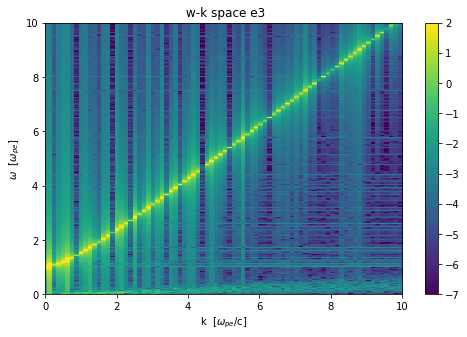• $\omega(k)$ with wavenumber in units of [k] = $\lambda_{De}$:
dirname = 'v02'
osiris.plot_wk(rundir=dirname, wlim=[0,10], klim=[0,0.4], vth = 0.02, vmin=-7, vmax=2, show_theory=True,
debye=True, plot_or=3)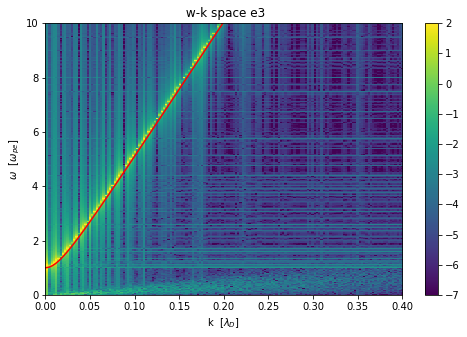I would like you to think about units:

• What do you think are the natural units for $\omega$?

We are plotting them in what are called normalized units (not inverse time or inverse distance). We use two choices ($\omega/\omega_p$ and $kc/\omega_p$) and ($\omega/\omega_p$ and $kv_{th}/\omega_p = k\lambda_D$).

We also plot the theory curve:

• Does it make sense?
• Why do you think it agrees better for all wavenumber than did the plot in Project 1a whichw as for Bohm-Gross waves?

## 3. Run a case in which  $vth_1=vth_2=vth_3=0.05 c$.

# vth/c = 0.05
dirname = 'v05'
osiris.runosiris(rundir=dirname,inputfile='v05.txt')

Make $\omega(k)$ plots for this case by running the cells below.

• $\omega(k)$ with wavenumber in units of [k] = $\omega_{pe}/c$:
dirname = 'v05'
osiris.plot_wk(rundir=dirname, wlim=[0,10], klim=[0,10], vth = 0.05, vmin=-7, vmax=2, plot_or=3)
osiris.plot_wk(rundir=dirname, wlim=[0,10], klim=[0,10], vth = 0.05, vmin=-7, vmax=2, plot_or=3, show_theory=True)• $\omega(k)$ with wavenumber in units of [k] = $\lambda_{De}$:
dirname = 'v05'
osiris.plot_wk(rundir=dirname, wlim=[0,10], klim=[0,0.4], vth = 0.05, vmin=-7, vmax=2, show_theory=True,
plot_or=3, debye=True)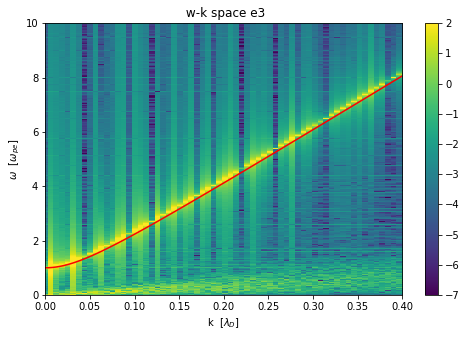### 3.1. Questions

• Do the $\omega(k)$ plots make sense?
• For which normalized units do the plots look similar to case b?

## 4. Run a case in which  $vth_1=vth_2=vth_3=0.20 c$.

# vth/c = 0.2
dirname = 'v20'
osiris.runosiris(rundir=dirname,inputfile='v20.txt')

Make $\omega(k)$ plots for this case by running the cells below.

• $\omega(k)$ with wavenumber in units of [k] = $\omega_{pe}/c$:
dirname = 'v20'
osiris.plot_wk(rundir=dirname, wlim=[0,10], klim=[0,10], vth = 0.20, vmin=-5, vmax=4, plot_or=3)
osiris.plot_wk(rundir=dirname, wlim=[0,10], klim=[0,10], vth = 0.20, vmin=-5, vmax=4, plot_or=3, show_theory=True)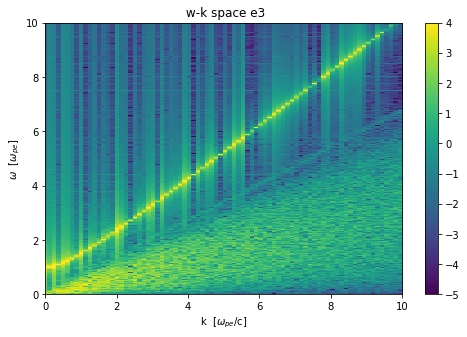• $\omega(k)$ with wavenumber in units of [k] = $\lambda_{De}$:
dirname = 'v20'
osiris.plot_wk(rundir=dirname, wlim=[0,10], klim=[0,0.4], vth = 0.20, vmin=-5, vmax=4, show_theory=True, plot_or=3, debye=True)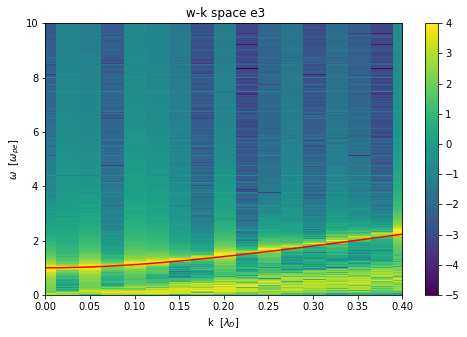### 4.1. Questions

• Do the $\omega(k)$ plots make sense?

• For which normalized units do the plots look similar to case a and b?

• Look closely at $k=0$. The frequency does not agree with theory as well as for cases a and b.

• Is it higher or lower than theory?

• Can you think of a reason why?

• Does the agreement with the theory depend much the temperature? Can you justify your answer using some equations?